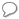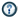xKinetic energyOverview

The kinetic energy of an object is the energy
Energy
In physics, energy is an indirectly observed quantity. It is often understood as the ability a physical system has to do work on other physical systems...

which it possesses due to its motion
Motion (physics)
In physics, motion is a change in position of an object with respect to time. Change in action is the result of an unbalanced force. Motion is typically described in terms of velocity, acceleration, displacement and time . An object's velocity cannot change unless it is acted upon by a force, as...

.
It is defined as the work needed to accelerate a body of a given mass from rest to its stated velocity
Velocity
In physics, velocity is speed in a given direction. Speed describes only how fast an object is moving, whereas velocity gives both the speed and direction of the object's motion. To have a constant velocity, an object must have a constant speed and motion in a constant direction. Constant ...

. Having gained this energy during its acceleration
Acceleration
In physics, acceleration is the rate of change of velocity with time. In one dimension, acceleration is the rate at which something speeds up or slows down. However, since velocity is a vector, acceleration describes the rate of change of both the magnitude and the direction of velocity. ...

, the body maintains this kinetic energy unless its speed changes. The same amount of work is done by the body in decelerating from its current speed to a state of rest.

The speed, and thus the kinetic energy of a single object is frame-dependent (relative): it can take any non-negative value, by choosing a suitable inertial frame of reference
Inertial frame of reference
In physics, an inertial frame of reference is a frame of reference that describes time homogeneously and space homogeneously, isotropically, and in a time-independent manner.All inertial frames are in a state of constant, rectilinear motion with respect to one another; they are not...

.DiscussionsSomething wrong with kinetic energyWhen a vehicle is traveling at sixty miles per hour does it have the potential or can it turn a generator by the wind which is produced by its` motion...Brainstorming ideas for floating helical hydraulic turbine hydro power stationsDoes anyone know any interesting facts about kinetic energy?IS THERE AN ENOUGH OF KINETIC ENERGY TO GO AROUND IN THE NEAR FUTUREDoes anyone know what kinetic energy can be converted to instead of electricity?